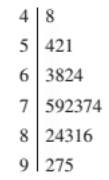Chapter 2, Problem 24PStatistics for The Behavioral Scie...

10th Edition
Frederick J Gravetter + 1 other
ISBN: 9781305504912

Solutions

Chapter
SectionStatistics for The Behavioral Scie...

10th Edition
Frederick J Gravetter + 1 other
ISBN: 9781305504912
Textbook Problem

A set of scores has been organized into the following stem and leaf display. For this set of scores: a. How many scores are in the 70s? b. Identify the individual scores in the 70s. c. How many scores are in the 40s? d. Identify the individual scores in the 40s.To determine

(a)

The number of scores in 70s.

Explanation

Given:

( 4 5 6 7 8 9 | 8 4,2,1 3,8,2,4

To determine

(b)

To identify:

The individual scores in the 70s.

To determine

(c)

The number of scores in 40s.

To determine

(d)

To identify:

The individual scores in40s.

Still sussing out bartleby?

Check out a sample textbook solution.

See a sample solution

The Solution to Your Study Problems

Bartleby provides explanations to thousands of textbook problems written by our experts, many with advanced degrees!

Get Started

In Exercises 1-6, simplify the expression. 11(1x)

Calculus: An Applied Approach (MindTap Course List)

For what value of k does the equation e2x=kx have exactly one solution?

Single Variable Calculus: Early Transcendentals, Volume I

In Exercises 7-12, refer to the following figure. 12. Which point has a y-coordinate that is equal to zero?

Applied Calculus for the Managerial, Life, and Social Sciences: A Brief Approach

The average value of f(x) = 3x2 + 1 on the interval [2, 4] is: a) 29 b) 66 c) 58 d) 36

Study Guide for Stewart's Single Variable Calculus: Early Transcendentals, 8th

In Exercises 2124, find the distance between the points. (1,3) and (4,9)

Finite Mathematics for the Managerial, Life, and Social Sciences